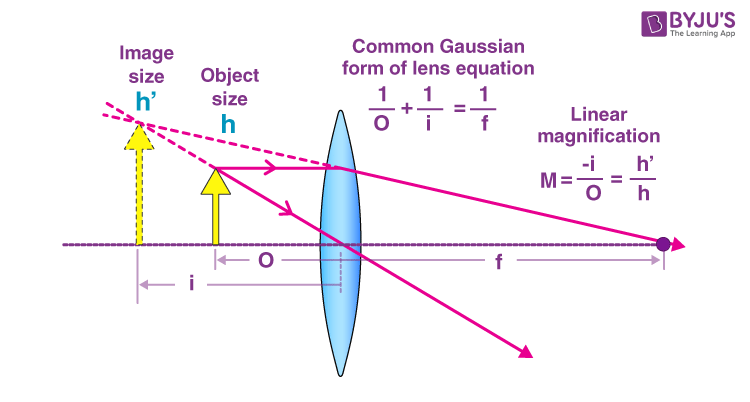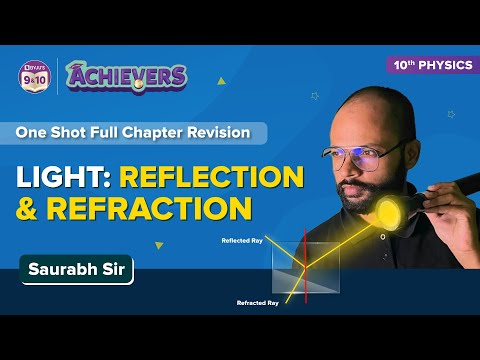# Lens Formula & Magnification - Lens Power

Spherical lenses are lenses formed by binding two spherical transparent surfaces together. There are two basic kinds of spherical lenses:

Concave lens: The lenses formed by binding two spherical surfaces such that they are curved inward are known as concave lenses.
Convex lens: The lenses formed by binding two spherical surfaces bulging outward are known as convex lenses.

## What Is Lens Formula?

Convex lenses are also known as converging lenses since the rays converge after falling on the convex lens, while concave lenses are known as diverging lenses as the rays diverge after falling on the concave lens. Images formed by these lenses can be real or virtual depending on their position from the lens and can have different sizes too. The image distance can be calculated with the knowledge of object distance and focal length with the help of the lens formula. In optics, the relationship between the distance of an image (i), the distance of an object (o), and the focal length (f) of the lens are given by the formula known as the Lens formula. The Lens formula is applicable for convex as well as concave lenses. These lenses have negligible thickness. It is an equation that relates the focal length, image distance, and object distance for a spherical mirror. It is given as,

$$\begin{array}{l} \frac{1}{i} + \frac{1}{o} = \frac{1}{f} \end{array}$$

i= distance of the image from the lens

o= distance of the object from the lens

f= focal length of the lensThe lens formula is applicable to all situations with appropriate sign conventions. This lens formula is applicable to both the concave and convex lenses. If the equation shows a negative image distance, then the image is a virtual image on the same side of the lens as the object. If this equation shows a negative focal length, then the lens is a diverging lens rather than the converging lens. This equation is used to find image distance for either real or virtual images.## Calculating magnification with the help of the lens formula:

The magnification of a lens is defined as the ratio of the height of an image to the height of an object. It is also given in terms of image distance and object distance. It is equal to the ratio of image distance to that of object distance.

$$\begin{array}{l} m = \frac{h’}{h} = \frac{v}{u} \end{array}$$

Where m= magnification

h’= height of the image

h = height of an object

v is the image distance

u is the object distance

### Power of Lens

The power of a lens is the measure of the degree of convergence or divergence of the light rays falling on it. The degree of convergence or divergence depends upon the focal length of the lens. Thus, we define the power of the lens as the reciprocal of the focal length of the lens used. It is given as,

1/f

Where f is the focal length of the lens used. SI unit of power is Dioptre (D). The power of the concave lens is negative, while the power of the convex lens can be positive.## Frequently Asked Questions – FAQs

Q1

### What is a convex lens?

Generally, an optical lens has two spherical surfaces. If the surface is bent or bulged outwards, then the lens is known as a convex lens.
Q2

### What is meant by the power of a lens?

The power of a lens is the measure of the degree of divergence or convergence of the light ray striking it. The degree of divergence or convergence relies on the lens’s focal length.
Q3

### What is the relationship between the focal length and the power of a lens?

The power of a lens is the reciprocal of the given lens’s focal length.
Q4

### What is the SI unit for the power of a lens?

Dioptre is the SI unit for calculating the power of a lens.
Q5

### What is meant by the focal length?

The focal length is the distance between a lens and the focal point. This is the length from a mirror or lens at which parallel light rays meet.

## Watch the video and revise all the important concepts in the chapter Light Reflection and Refraction Class 10Stay tuned with BYJU’S to learn more about lens formula, magnification, and the power of the lens.

Test your knowledge on Lens formula